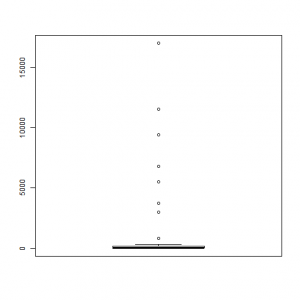Want to share your content on R-bloggers? click here if you have a blog, or here if you don't.One of the first steps of data analysis is the descriptive analysis; this helps to understand how the data is distributed and provides important information for further steps. This set of exercises will include functions useful for one variable descriptive analysis, including graphs. Before proceeding, it might be helpful to look over the help pages for the `length, range, median, IQR`, `hist`, `quantile`, `boxplot`, and `stem` functions.

For this set of exercises you will use a dataset called `islands`, an R dataset that contains the areas of the world’s major landmasses expressed in squared miles. To load the dataset run the following instruction: `data(islands)`.

Answers to the exercises are available here.

If you obtained a different (correct) answer than those listed on the solutions page, please feel free to post your answer as a comment on that page.

Exercise 1

Load the `islands` dataset and obtain the total number of observations.

Exercise 2

Measures of central tendency. Obtain the following statistics of islands

a)Mean
b)Median

Exercise 3

Using the function `range`, obtain the following values:

a)Size of the biggest island
b)Size of the smallest island

Exercise 4

Measures of dispersion. Find the following values for islands:

a)Standard deviation
b)The range of the islands size using the function `range`.

Exercise 5

Quantiles. Using the function `quantile` obtain a vector including the following quantiles:

a) 0%, 25%, 50%, 75%, 100%
b) .05%, 95%

Exercise 6

Interquartile range. Find the interquartile range of islands.

Exercise 7

Create an histogram of islands with the following properties.

a) Showing the frequency of each group
b) Showing the proportion of each group

Exercise 8

Create box-plots with the following conditions

a) Including outiers
b) Without outliers

Exercise 9

Using the function `boxplot` find the outliers of islands. Hint: use the argument `prob=F`.

Exercise 10

Create a stem and leaf plot of islands